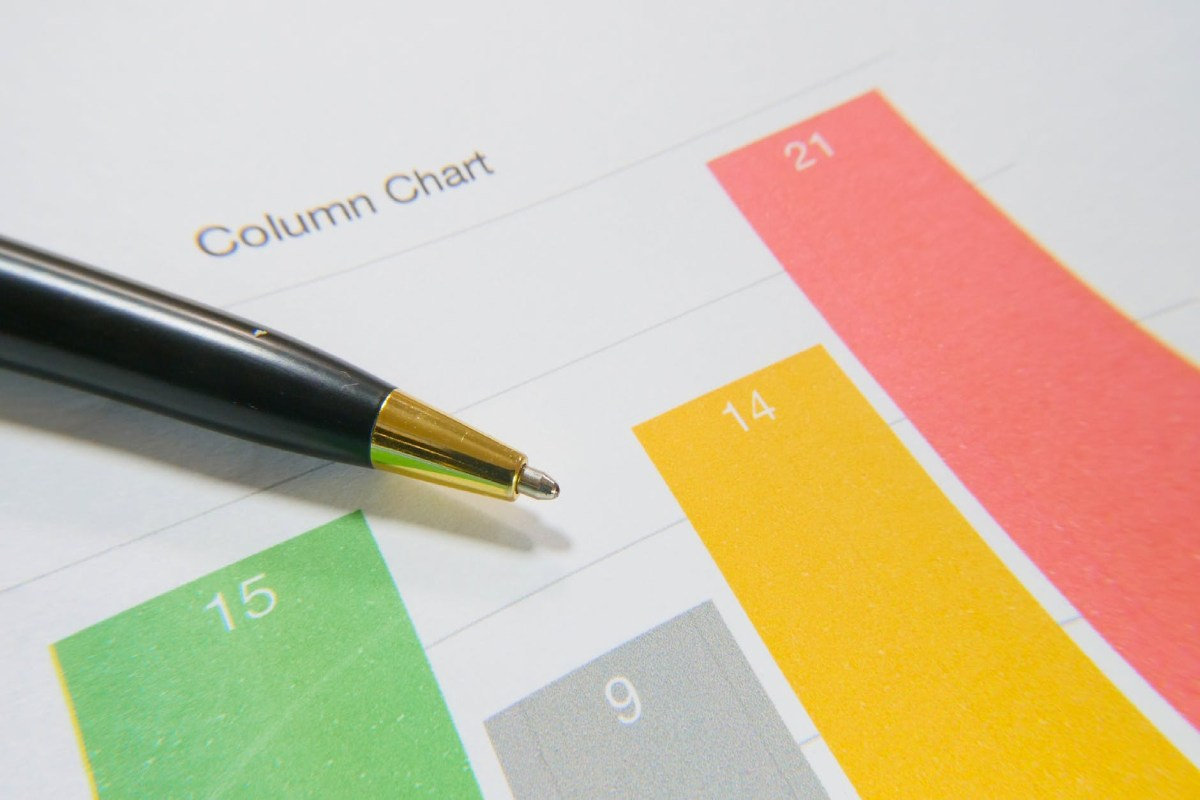September 23, 2023

# How to Have a Good Command on Bar Graphs?Others

Bar graphs are the graphs that represent the data in pictorial form, displayed vertically or horizontally as rectangle-shaped bars, the bars have a length that is proportional to the measurement of data. Bar graphs are famously known as bar charts and they are the only means of handling the data in statistics.

The interpretation, organization, presentation, observations, and analysis of data are termed statistics. There are several methods to represent the data in statistics like tables, frequency polygons, pie charts, bar graphs, histograms, etc. Let us now discuss what a bar chart means, what are its different types, and what its uses.

The bars that are drawn in the graphs are of even width, the representation of a variable quantity is done on-axis and the depiction of the measure of the variable is done on the other axis. The value of the variables is denoted by the lengths and heights of bars. The graphs are also useful for the comparison of certain quantities.

## Three Main Characteristics of Bar Charts:

• The bar charts help in the comparison of different data sets amongst a different set of groups very easily.
• It demonstrates the relationship between two axes, one axis has the categories and the other discrete values.
• Over time the key changes in data on the graph are displayed.

### We have four types of Bar Charts:

#### Vertical Charts

These are represented vertically on the graph using bars; the bars signify the data measure. The height that these bars have, displays the values, and data representation is done on the y-axis.

#### Horizontal Charts

These are represented horizontally on the graph using bars; the bars signify the data measure. The length that these bars have, displays the values, and data representation is done on the x-axis.

#### Clustered Bar Charts

The clustered bar chart is also known as a grouped bar chart, which represents the distinct value for objects that are in the same category. In these types of charts, the entire number of illustrations is displayed in a single bar after combining. A grouped bar chart compares various data sets. Here, a solo color is used for the representation of a precise series across the data set. The representation of this graph can be done using horizontal and vertical bar graphs.

#### Composite Bar Charts

The composite bar chart is also known as the stacked bar chart; this divides the total into various parts. The representation in these kinds of graphs is done using various colors, because of which different categories can be easily identified. These charts require precise labeling to display the diverse parts in the bar.

## There are a few important properties of a Bar Chart, let’s take a quick look at them:

• A common base is very important for all the bars.
• Each and every column should have equal width in the Bar Chart.
• The height and data value of the bar should be corresponding.
• The distance must be the same amongst each bar.

• This line graph presentation can summarise huge amounts of data in simple pictorial form.
• Each category of the data is displayed in a frequency distribution.
• It explains the tendency of data far better than tables.
• It assists in approximating the important values at a glance.

• At times, the bar charts fail to disclose the cause, patterns, and effects.
• It can be manipulated easily to yield fake information.

### Few tips for drawing a Bar Chart:

We have the data as pets like a kitten, dog, bunny, and hamster and the numbers that correspond are 25, 30, 5, and 8.

1. Deciding the Title of the Bar Chart.
2. Naming or labeling the horizontal axis, with pet names.
3. Now, label the vertical axis with the Numbers corresponding to the pets.
4. Finally, draw the Bar Chart representing the data and finalize the scale.

Cuemath provides various explanations with examples online for a better understanding of the subject, refer to them and clear all your doubts.